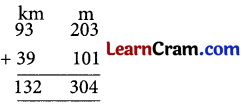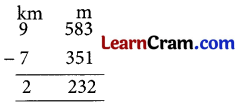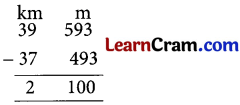# DAV Class 3 Maths Chapter 8 Worksheet 3 Solutions

The DAV Class 3 Maths Solutions and DAV Class 3 Maths Chapter 8 Worksheet 3 Solutions of Length offer comprehensive answers to textbook questions.

## DAV Class 3 Maths Ch 8 WS 3 Solutions

Question 1.

(a) 79 km 685 m and 35 km 214 m.
Solution:(b) 7 km 326 m and 9 km 534 m.
Solution:(c) 11 km 356 m and 32 km 434 m.
Solution:(d) 93 km 203 m and 39 km 101 m.
Solution:Question 2.
Arrange in columns and subtract.

(a) 7 km 351 m from 9 km 583 m.
Solution:(b) 32 km 141 m from 75 km 392 m.
Solution:(c) 37 km 493 m from 39 km 593 m.
Solution:(d) 41 km 743 m from 73 km 854 m.
Solution:### DAV Class 3 Maths Chapter 8 Worksheet 3 Notes

Question 1.
Add 50 km 420 m and 20 km 430 m
Solution: